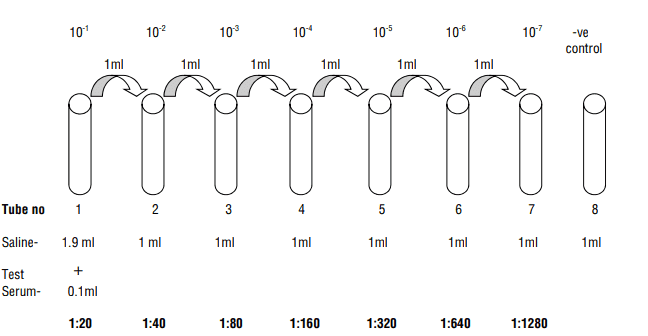# Serial dilutionThis snip off is from Himedia Widal test teaching kit.

I understand 1/20 1/40 1/80... are the concentrations in each test tube. They are obtained by the following calculation: (2nd testube) 1/2 X 1/20.
But I can't decipher the numbers written at the top, 10-1 , 10-2 etc.

Besides which of of these two set of numbers should be called dilution factor.

#### Attachments

mfb
Mentor
That should be ##2^{-n}## for different n. Maybe it was copied from a different problem where the dilution was a factor 10 each step.

•TytoAlba95
Baluncore
2021 Award
Welcome to PF.
I think they have used old numbers from steps of 0.1 when they should now use steps of 0.5
Following the dilution, the concentration in the tubes will be;
#1, 1:20 = 0.05 = 0.1 × 2-1
#2, 1:40 = 0.025 = 0.1 × 2-2
#3, 1:80 = 0.0125 = 0.1 × 2-3
#4, 1:160 = 0.00625 = 0.1 × 2-4
#5, 1:320 = 0.003125 = 0.1 × 2-5
#6, 1:640 = 0.0015625 = 0.1 × 2-6
#7, 1:1280 = 0.00078125 = 0.1 × 2-7
In each case the 0.1 = 10-1 = initial dilution of 10.

•TytoAlba95
Which number here could be called dilution factor?

Thanks

Baluncore
2021 Award
The dilution factor is equal to the final volume divided by the aliquot volume.
For tube #1; 1:20; The dilution factor is 2.0 mL / 0.1 mL = 20.
The dilution factors for tubes #1 to #7 are 20, 40, 80, 160, 320, 640 and 1280.

•TytoAlba95
The dilution factor is equal to the final volume divided by the aliquot volume.
Thanks

Merlin3189
Homework Helper
Gold Member
•